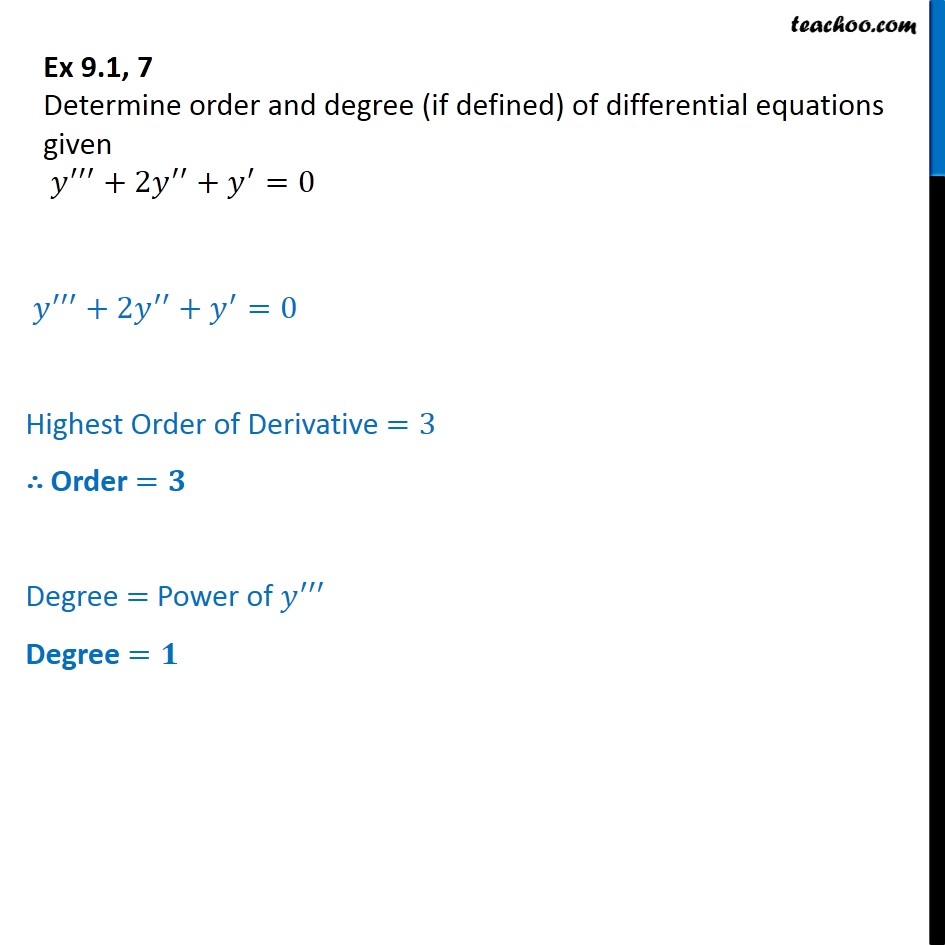Ex 9.1

Chapter 9 Class 12 Differential Equations
Serial order wiseGet live Maths 1-on-1 Classs - Class 6 to 12

### Transcript

Ex 9.1, 7 Determine order and degree (if defined) of differential equations given y +2y + y =0 y +2y + y =0 Highest Order of Derivative =3 Order = Degree = Power of y Degree =